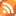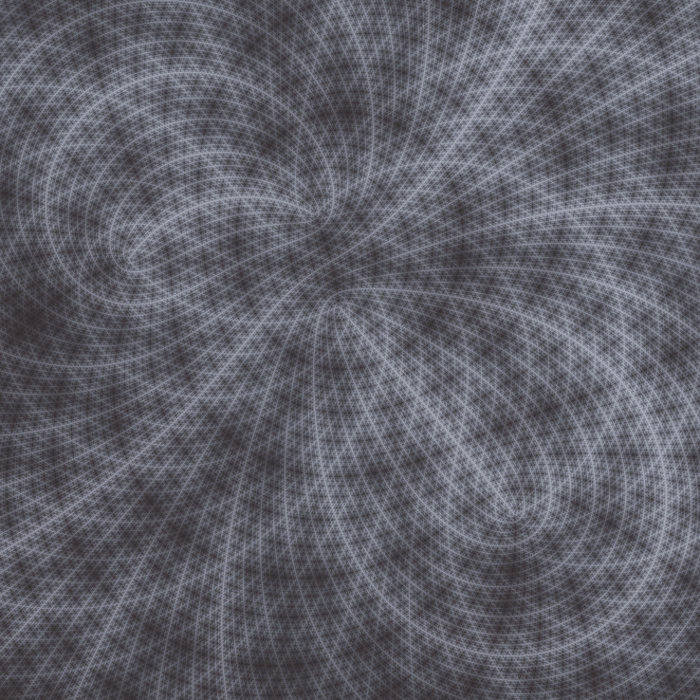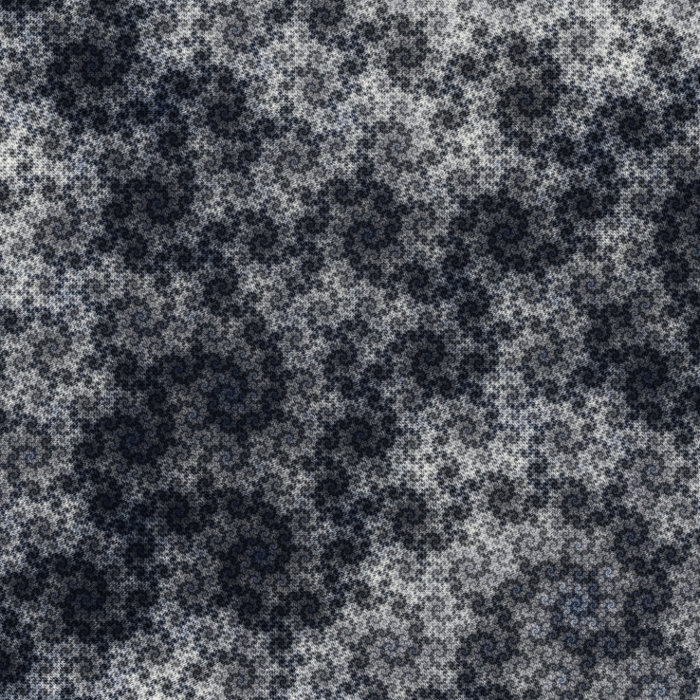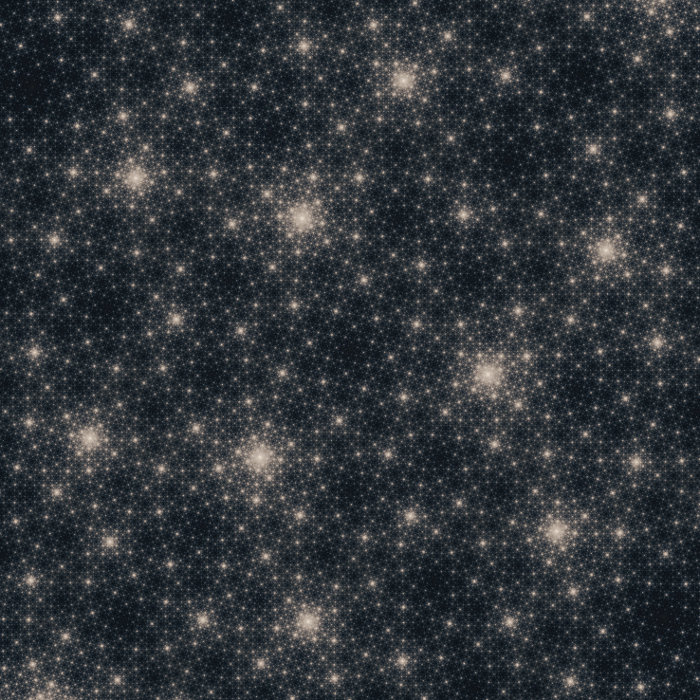# Algorithmic worlds

## -

### Search blog posts

Date:

Tags:

Next posts

2013-06-09 Variations on Nova

2013-05-12 Two new gigapixel images

2013-04-28 Lattes Julia sets

2012-10-21 3d hyperbolic limit sets

2012-07-01 Fractal sea creatures

2012-06-24 Nicholas A. Cope

2012-06-16 3d printed Julia set

2012-05-06 Pep Ventosa

2012-04-06 Fractal automata

2012-03-30 Periodic Julia patterns

# BlogApril 28th 2013

## Lattès Julia sets

In a previous post, we saw how the fact that the torus can be realized as a branched covering of the sphere allowed one to draw periodic Julia patterns. Today, I'd like to speak about another application of this fact, yielding the first dense Julia sets to have been recognized as such, by the french mathematician Samuel Lattès in 1918. This post will be a bit mathematical. I'll do my best to be pedagogical, with no guarantees. For readers interested, I explain at the end of this post how to visualize concretely the branched covering of the sphere by a torus.

Recall that Julia sets arise as iterations of conformal maps of the sphere to itself. There are essentially two types of orbits. Either an orbit will stay close to the neighbouring orbits, in which case the point is said to be regular, or the orbit is chaotic and the neighbouring orbits tend to get far apart. In the latter case, the point is said to belong to the Julia set. We obtain in this way a subset of the sphere. Dense Julia sets cover the whole sphere, which means that all the orbits are chaotic. A more detailed discussion can be found in this blog post. How can we construct such maps?

A very neat way is to use the branched covering of the sphere by a torus. The torus can be seen as a periodically identified complex plane, in other words the quotient of the complex plane by a two-dimensional lattice. This makes it very easy to create chaotic maps on the torus. Just take an affine map A(z) = az + b of the complex plane to itself mapping the lattice to itself. If the map is expanding, i.e. if |a| > 1, it is easy to see that after sufficiently many iterations, any two points will get far apart from each other on the torus.

How does this help creating chaotic maps on the sphere? Suppose that you can find a map F of the sphere to itself which is covered by an affine map of the torus. By this, I mean the following. Let p: T^2 -> S^2 be the covering map of the sphere by the torus. Let w be a point of the torus such that p(w) = z. Then the map F is covered by the affine map A if F(z) = p(A(w)), and if this is true for any w such that p(w) = z. The expanding property of the torus map is shared by F, as a consequence of which all the orbits of F are chaotic. Such conformal maps F of the sphere are called Lattès maps.

To visualize these Julia sets, we have to assign a color to each orbit according to some of its characteristics. A convenient way is to use some version of the orbit trap method, see this blog post. Here are three views of Lattès Julia sets. Click for high resolution zoomable images!20120329-1. A dense Julia set constructed from iterations of the following Lattès map: z -> (z2-a)2/(4z(z-1)(z-a)), a being a complex parameter.20120407-1. A dense Julia set constructed from iterations of the following Lattès map: z -> (z + 1/z)/2i.20120601-1. A dense Julia set constructed from iterations of the following Lattès map: z -> (z3+a)/(az3+1), with a = exp(2pi/3)

If you look carefully, you can see hints that the conformal map is coming from a torus. For instance, all the structures (dots, centers of spirals) seem to be aligned on a conformally distorted grid. This is a hint of the lattice underlying the covering torus. Many more pictures of Lattès Julia sets can be found in this collection. The corresponding Lattès maps are indicated below each picture.

For more information about Lattès maps, check this paper by Milnor. Explicit formulas can be found near the end. If you are not familiar with complex dynamical systems, these lectures may help.

And as promised, for the interested reader, here are some explanations about how to visualize the covering of the sphere by a torus (actually this is only the simplest one, there are several such coverings). In the following, it will be very useful if you take some piece of paper to draw what I'm going to explain. First, you have to be familiar with the representation of a torus by a rectangle (or a parallelogram) whose opposide sides are identified two by two.

So to get a sphere out of this torus, do the following. Pick the center of the rectangle (we consider a rectangle to keep things a bit simpler), and identify the points opposite from each other with respect to the center. If you are careful about the identification of the opposite sides, you will see that, in addition to the center itself, there are three other points that are not identified with any other (or rather their image opposite to the center is themselves). They form the four banching points of the covering. Now you can see that any point of the rectangle either lies on the left side half of the rectangle, or is identified with a point lying there. What remains to do is to convince yourself that this half-rectangle, together with the original identifications coming from the torus and the new identification we added, is really a sphere.